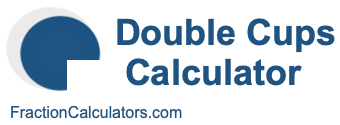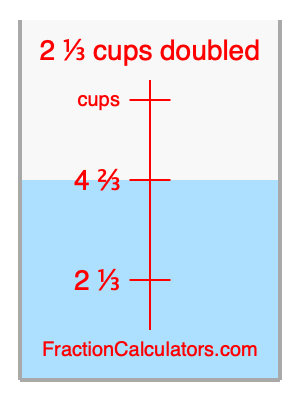What is 2 1/3 cups doubled?Here we will calculate 2 1/3 cups doubled and illustrate 2 1/3 cups doubled on a measuring cup. What is 2 1/3 cups doubled? 2 1/3 cups doubled is twice 2 1/3 cups. Therefore, to double 2 1/3 cups, we multiply 2 1/3 by 2. Here is the formula, the math, and the answer to 2 1/3 cups doubled:

Cup × 2 = Cup doubled
2 1/3 × 2 = 4 2/3
2 1/3 cups doubled = 4 2/3 cups

Below is an illustration of a measuring cup. We show 2 1/3 cups and 2 1/3 cups doubled (4 2/3 cups) so you can see where they are in relation to each other.As you can see, we filled up our measuring cup to 2 1/3 cups doubled. In other words, we filled it up to 4 2/3, which is two times 2 1/3.

Double Cups Calculator
Here you can double another cup measurement.

What is cups doubled?

What is 2 3/8 doubled?
Here is the next measurement on our list that we have doubled for you.